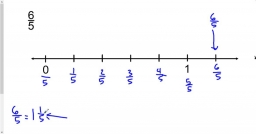# Two-digit 7607

The digit sum of a two-digit number is 11. After changing the order of the digits, I get a number 27, smaller than the intended number. What number do I think?

x =  74

### Step-by-step explanation:Did you find an error or inaccuracy? Feel free to write us. Thank you!

Tips for related online calculators
Do you solve Diofant problems and looking for a calculator of Diofant integer equations?